# When Are Triangles Similar? (3 Ways To Tell, With Examples)

In geometry, you will often compare triangles to see if their side lengths or angles are the same.  Congruent triangles are exactly the same in terms of side lengths and angles – but what about similar triangles?

So, when are triangles similar?  Two triangles are similar when each triangle has the same set of angle measures. For example, if Triangle A has angle measures of 20, 50, and 110 degrees, then Triangle B is similar to Triangle A if and only if its angle measures are 20, 50, and 110 degrees.

Of course, we can use several different criteria to determine when two triangles are similar.

In this article, we’ll talk about similar triangles and how to tell when triangles are similar.  We’ll also look at several examples to make the concept clear in various scenarios.

Let’s get started.

## When Are Triangles Similar?

Two triangles are similar when each triangle has the exact same set of three angle measures.  For example:

• If Triangle A has angle measures of 30, 70, and 80 degrees, and Triangles A and B are similar, then Triangle B must also have angle measures of 30, 70, and 80 degrees.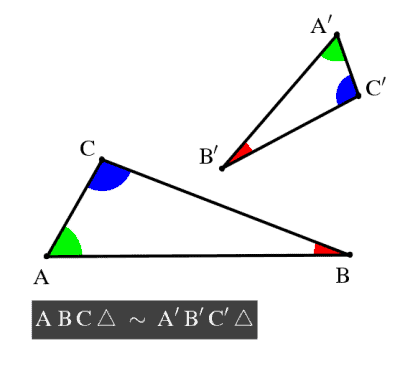Two triangles are similar if their three pairs of angles match up with the exact same angle measures.

For similar triangles, each side length of one triangle is proportional to the corresponding side length of the other triangle.  For example:

• If Triangle A has side lengths 3, 4, and 5 feet, and Triangle B has side lengths 6, 8, and 10, then Triangles A and B are similar, since corresponding sides have proportion 2 (6 = 2*3, 8 = 2*4, 10 = 2*5).

The proportion between two similar triangles does not need to be a whole number.  The proportion could be any positive number P.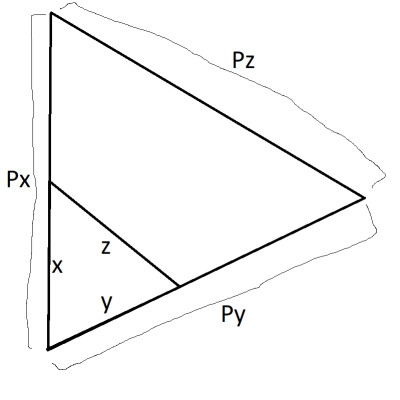The proportion between the side lengths of two similar triangles can be any positive number P.

If Triangle A has side lengths x, y, and z, then a similar triangle B would have side lengths Px, Py, and Pz.  Note that the area of Triangle B would be P2 times the area of Triangle A:

• Area of Triangle = (Base*Height)/2

Remember that a pair of congruent triangles is similar, and the corresponding side lengths are also the same.

Note: two triangles can be similar without being congruent.  In other words:

“If two triangles are congruent, then they are also similar.  However, two similar triangles may or may not be congruent.”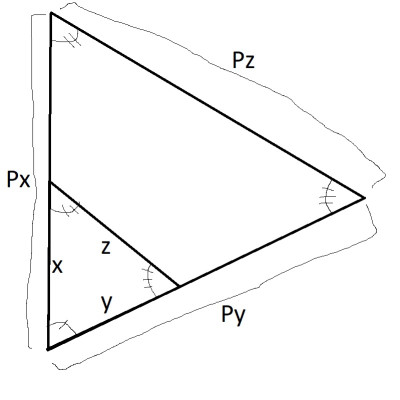Two triangles can be similar (same pairs of angles) but not congruent (different but proportional side lengths).

It all comes down to the proportion P between the corresponding side lengths.  For two similar triangles with proportion P between corresponding side lengths:

• If P = 1, then the two similar triangles are congruent (they have the same size).
• If P is not 1, then the two similar triangles are not congruent (they do not have the same size).

## How To Prove That Two Triangles Are Similar

There are several situations to look out for that will help you to prove that two triangles are similar:

### AA (Angle-Angle)

AA stands for “angle-angle”.  When two corresponding pairs of angles in a triangle have the same measure, then the third pair of angles must also have the same measure.

This means that the two triangles are similar, since all three pairs of angles have the same measure.  This is because the angles in any triangle add up to 180 degrees.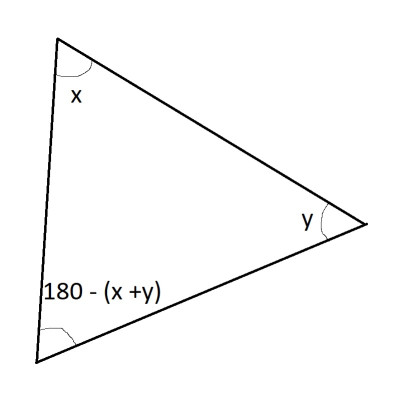The three angles in any triangle add up to 180 degrees. If we know the angle measures of two angles (x and y), then we know that the measure of the third angle is 180 – (x + y).

If Triangle A has angles a, b, and c, while Triangle B has angles x, y, and z, with two angles matching (a = x and b = y), then:

• 180 = 180
• a + b + c = x + y + z  [sum of the three angles is 180 in any triangle]
• x + y + c = x + y + z  [a = x and b = y]
• c = z

So the third pair of angles match, and the triangles are similar.

#### Example: Similar Triangles By AA (Angle-Angle)

Let’s say that:

• Triangle A has angle measures 20 degrees and 40 degrees
• Triangle B has angle measures 20 degrees and 40 degrees

Then Triangles A and B are similar by AA (angle-angle).  We can prove that the third angle is 120 degrees, since:

• a + b + c = 180
• 20 + 40 + c = 180
• 60 + c = 180
• c = 120

So both triangles have angle measures of 20, 40, and 120 degrees.  Thus, A and B are similar triangles.

### SAS (Side-Angle-Side)

SAS stands for “side-angle-side”.  When two corresponding pairs of sides in a triangle are proportional (with the same proportion) and one corresponding pair of angles (the ones between the two sides) has the same measure, then the triangles are similar.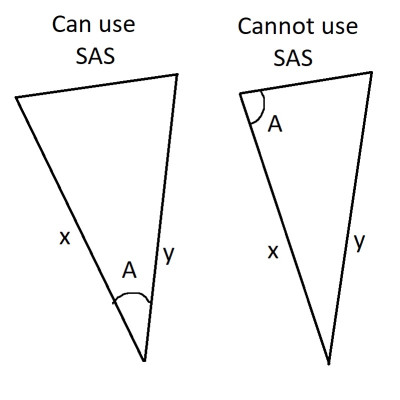To use SAS (side-angle-side) to prove triangle similarity, the known angle (A) must be “included” (between the two known sides).

Think about taking two lines of length x and y, and putting them together at the ends so that you have a fixed angle A between them.  Take another two lines of lengths Px and Py and put them together at the ends so that they have the same angle A between them.

The third side of the triangles have proportional length (z and Pz), which means the triangles are similar.

We can also prove similarity using the Law of Cosines. Let’s say we have the two triangles arranged so that their shared angle meets at the same point (as shown in the diagram below):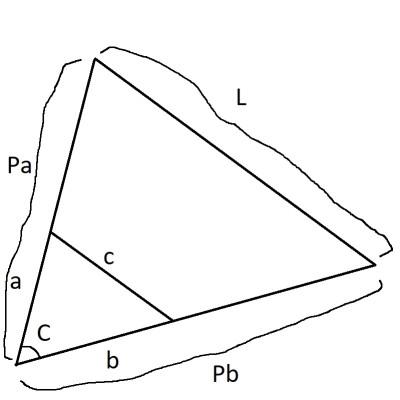The two triangles are arranged so that they meet at their common (shared) angle, C. The small triangle has side lengths a, b, and c, while the larger triangle has side lengths Pa, Pb, and L.

These triangles satisfy SAS, since the side pairs a/Pa and b/Pb are proportional (with the same proportion P), and they share the included angle C.

For the smaller triangle, the Law of Cosines says that:

• a2 + b2 – 2abcos(C) = c2

When we apply this formula to the larger triangle, we get:

• (Pa)2 + (Pb)2 – 2(Pa)(Pb)cos(C) = L2

After simplifying, we get:

• P2a2 + P2b2 – 2P2abcos(C) = L2

Note that P2 appears in every term on the left side of the equation.

After dividing by P2 on both sides, we get:

• a2 + b2 – 2abcos(C) = L2/P2

From the first equation above, we know that a2 + b2 – 2abcos(C) = c2, so:

• c2 = L2/P2
• c2P2 = L2
• cP = L

So, the sides c and L have the same proportion of P as the sides a/Pa and b/Pb.

Thus, the two triangles are similar.

#### Example: Similar Triangles By SAS (Side-Angle-Side)

Let’s say that:

• Triangle A has two side lengths 3 and 4, with an angle of 30 degrees between them.
• Triangle B has two side lengths 6 and 8, with an angle of 30 degrees between them.

Then Triangles A and B are similar by SAS (side-angle-side):

• The pairs of sides are proportional with P = 2, since 6 = 2*3 and 8 = 2*4.
• The angle between the sides is 30 degrees in both triangles.

### SSS (Side-Side-Side)

SSS stands for “side-side-side”.  When three corresponding pairs of sides in a triangle have proportional length (with the same proportion), then the triangles are similar.

The proof would again use the Law of Cosines. With side lengths a, b, and c for one triangle and side lengths Pa, Pb, and Pc for the other triangle, we would see that both angles in each corresponding pair have the same degree measure.

#### Example: Similar Triangles By SSS (Side-Side-Side)

Let’s say that:

• Triangle A has side lengths 2, 5, and 6.
• Triangle B has side lengths 8, 20, and 24.

Then Triangles A and B are similar by SSS (side-side-side):

• The pairs of sides are proportional with P = 4, since 8 = 2*4, 20 = 5*4, and 24 = 6*4.

### HL (Hypotenuse-Leg)

HL stands for hypotenuse-leg.  In the special case of right triangles, we can prove similar triangles if the hypotenuses are proportional and one pair of legs are proportional (with the same proportion).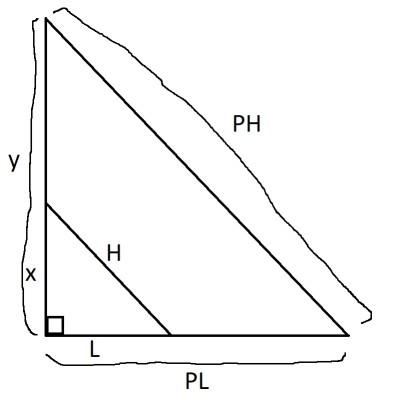The two right triangles are arranged so that they meet at their common (shared) angle, the right angle of 90 degrees. The small triangle has side legs x and L with hypotenuse H, while the larger triangle has side legs y and PL, with hypotenuse PH.

For the smaller right triangle, the Pythagorean Theorem applies:

• x2 + L2 = H2

Solving for x2, we get:

• x2 = H2 – L2

Applying the Pythagorean Theorem to the larger right triangle, we get:

• y2 + (PL)2 = (PH)2
• y2 + P2L2 = P2H2
• y2 = P2H2 – P2L2

Note that P2 appears in every term on the right side of the equation.

After dividing by P2 on both sides, we get:

• y2/P2 = H2 – L2

From the first equation above, we know that x2 = H2 – L2, so:

• y2/P2 = x2
• y2 = P2x2
• y = Px

So, the sides y and x have the same proportion of P as the sides L/PL and H/PH.

Thus, the two triangles are similar.

#### Example: Similar Triangles By HL (Hypotenuse-Leg)

Let’s say that:

• Right Triangle A has a hypotenuse of length 10 and a leg of length 6.
• Right Triangle B has a hypotenuse of length 50 and a leg of length 30.

Then Triangles A and B are similar by HL (hypotenuse-leg):

• The pair of hypotenuses and the pair of sides are proportional with P = 5, since 50 = 10*5 and 30 = 6*5.

### Equilateral Triangles

Any two equilateral triangles are automatically similar.  Remember that for any equilateral triangle, all three angles have the same measure of 60 degrees.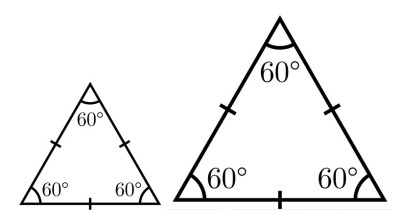Two equilateral triangles are automatically similar (same angles and proportions between side lengths). However, they may not be congruent, due to different sizes (side lengths).

So, each pair of angles between two equilateral triangles matches up (both are 60 degrees).  Remember that two equilateral triangles are similar, but they may or may not be congruent (the side lengths may differ).

## Transitivity Of Similarity Of Triangles

Remember that similarity of triangles is transitive.  That is:

“If Triangle A is similar to Triangle B and Triangle B is similar to Triangle C, then Triangle A is similar to Triangle C.”

Furthermore, we can find the proportion between triangles A and C.  If:

• Triangle A’s side lengths are P times Triangle B’s side lengths, and
• Triangle B’s side lengths are Q times Triangle C’s side lengths, then
• Triangle A’s side lengths are PQ times Triangle C’s side lengths.

## Conclusion

Now you know when triangles are similar and how to tell.  You also know several key scenarios (AA, SAS, SSS, HL, and equilateral triangles) and when triangles are also congruent.

You can learn how to find the perimeter of a triangle in various scenarios here.

You can learn about uses of triangles in real life here.